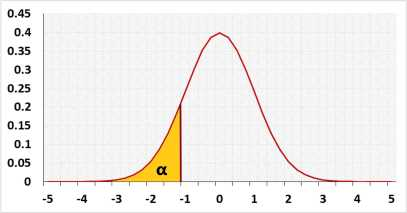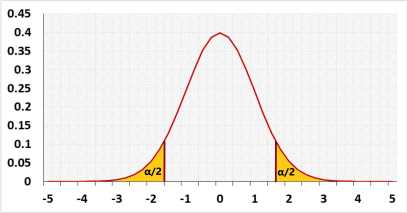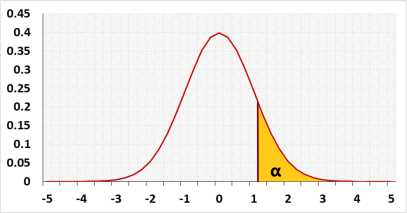# Wilcoxon Signed-Rank test

Two dependent samples, non-parametric paired test
The test compares the probability to get higher value from group1 with the probability to get higher value from dependent group2.

## Test calculation

Enter raw data directly
Enter raw data from excel

## Enter sample data

Header: You may change groups' name to the real names.
Data: When entering data, press Enter, , or Space after each value.
Difference: Difference = Right column - Left column.

 Before After

The tool ignores empty cells or non-numeric cells.

## Enter sample data

You may copy data from Excel, Google sheets or any tool that separate data with Tab and Line Feed.
Copy the data, one block of 3 consecutive columns includes the header, and paste below.
Copy the data,It is okay to leave empty cells, empty cells or non numeric cells won't be counted

### Results

Skewness Shape:

The difference between the expected SD and the sample SD

validation message

## Information

Hypotheses
H0: Before = After
H1: Before < > After
Test statisticNormal distributionRight tail, or two tails with positive Z, (W- > μ) , C = -0.5 .
Left tail, or two tails with negative Z, (W- < μ) , C = 0.5 .

### Target

Unlike paired t-test that compares the mean of the differences to zero, Wilcoxon Signed-Rank test compares the probability that a random value from Group1 (like before) is greater than his dependent value from Group2 (like after).
When the two distributions have a similar shape you can use the test to compare also the median of the differences to zero.
When the two distributions have a similar symmetrical shape, you can use the test to compare the mean of the differences to zero. more

### Method

Automatic - when n1≤20 and n2≤20 and the data doesn't have ties, the tool uses the exact value, otherwise the tool uses the z approximation.
Exact - when n1≤20 and n2≤20 the tool uses the exact value, calculated base on all the possible combinations, otherwise the tool uses the z approximation.
Z approximation - the tool uses the z approximations.

Continuity correction - relevant only for the Z approximation. It is used since a continuous distribution is used to calculate a discrete distribution.

The page was updated on April 2020 for a full exact distribution (n ≤ 40), instead of a common significant level table.

## R Code

The following R code should produce similar results:

The R code doesn't exclude outliers.